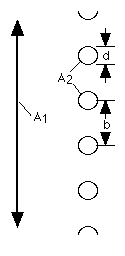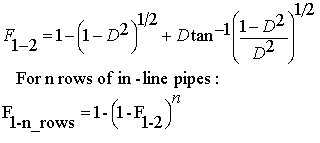# Problem 2833. Radiation Heat Transfer — View Factors (5)

View factors (aka configuration factors) are utilized in some radiation heat transfer models to estimate heat transfer rates between surfaces. In particular, the thermal energy leaving a given surface is applied to other surfaces, as appropriate, based on how much the hot surface "sees" the other surfaces. As such, view factors are purely geometrical in nature. A range of view factor formulae are available here.

For this problem, calculate the view factor from surface 1 (an infinitely long plate) to surfaces 2 (n rows of in-line pipes):The view factor for one row of pipes is F_1-2 (the first equation):The second equation is utilized for more than one row of pipes. Any of the variables can be a vector. Also, note that D = d/b, where d is the pipe diameter and b is the center-to-center spacing between pipes.

Note: This problem is identical to the previous problem (4) except that the variables will be provided in vectors of varying sizes. The variables will need to be combined to produce all the applicable combinations. For example, if the sizes of d, b, and n are 2, 5, and 1, respectively, there will be ten total variable combinations (and answers). As another example, if the sizes of d, b, and n are 4, 3, and 2, respectively, there will be 24 total variable combinations. This simulates a parametric design study. Also, because all possible combinations can be generated in various orders, the answers will be sorted; the order that you output answers does not matter.

### Solution Stats

42.05% Correct | 57.95% Incorrect
Last Solution submitted on Jul 26, 2023

### Community Treasure Hunt

Find the treasures in MATLAB Central and discover how the community can help you!

Start Hunting!